Home > CCG > Chapter 11 > Lesson 11.1.1 > Problem11-11

11-11.

For each of the relationships represented in the diagrams below, write and solve an equation for $x$ and/or $y$. Justify your method. In part (a), assume that $C$ is the center of the circle.

1.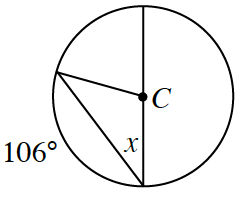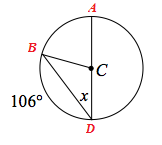$m\overarc{ABD}=180°$ because $\overline{AD}$ is a diameter

$m\overarc{AB} + m\overarc{BD} = m\overarc{ABD}$

$106º+m\overarc{BD}=180°$

$m\overarc{BD}=74°$

The measure of the intercepted arc is twice the measure of the inscribed angle

$\begin{array}{l} m\overarc{BD} = 2(x)\\ \phantom{000}74° = 2x\\ \phantom{0000}x=37° \end{array}$

One equation could be $2x=180°-106°$

1.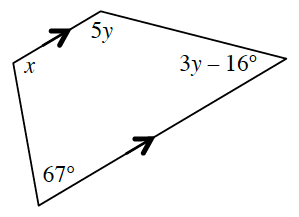Which angles are supplementary? This will help you write equations to solve for $x$ and $y$.

1.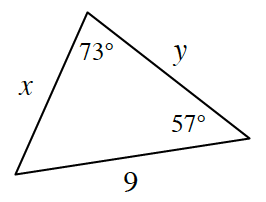Use the Law of Sines.

$x = 7.9$, $y = 7.2$

1.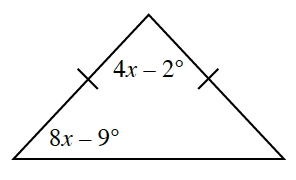Use the Triangle Angle Sum Theorem.